# How do you find the measure of each interior angle of a regular polygon?Abdullah

Solution:

Step:1 A regular polygon is defined as three or more sides having two-dimensional figure with equal magnitude of all interior angles and consequently all exterior angles.

Each interior angle of a regular polygon can be calculated by the formula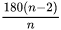, where n is the number of sides and formula is applicable for n greater than or equal to 3 sides.

Step:2 The formula is obtained by observing the pattern in three or more sided polygon sides and their sum of all interior angles.

The sum of all interior angles of the polygon is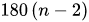, where n is the number of sides and formula such that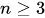.

For regular polygon, each sides and each interior angles are equal.

Step:3  Therefore, the measure of each interior of n-sided regular polygon can be calculated by dividing the total sum of interior angles from total number of sides.

So, each interior angle of n-sided polygon isStep:4 For example, each interior angle of a hexagon can be calculated by substituting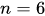in the above formula.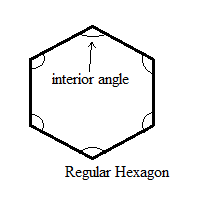Therefore, each interior angle of a regular hexagon is equal to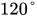.

Simillarly, each interior angle of a regular quadrilateral can be calculated by substituting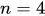in the formula.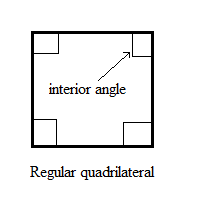Therefore, each interior angle of a regular quadrilateral is equal to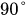.

Answer: The Each interior angle of a regular polygon can be calculated by the formula, where n is the number of sides.

Similar Problems:

Problem 1: Find the sum of interior angles of a pentagon.

Solution:

Step:1 The pentagon has five sides. It is a 5 sided polygon.

Step:2 The sum of all interior angles of the polygon is, where n is the number of sides and formula such that.

Step:3 Substitute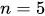in the formulaand obtain the sum of interior angles of a pentagon.Therefore, the sum of interior angles of a pentagon is 540 degree.

The sum of interior angles of a pentagon is 540 degree.

Problem 2: Find each interior angles of a regualr pentagon.

Solution:

Step:1 The pentagon has five sides. It is a 5 sided polygon.

Step:2 Each interior angle of a regular polygon can be calculated by the formula, where n is the number of sides and formula is applicable for n greater than or equal to 3 sides.

Step:3 Substitutein the formulaand obtain the each of interior angles of a regular pentagon.Therefore, each interior angles of a regular pentagon is equal to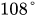.

The each interior angles of a regular pentagon is equal to.

### Need homework help now?

tutors online

Related Questions
##### (t) in °C of the pot of soup at time t (in The initial temperature of a pot of soup is 80°C. The temperature minutes) satisfies the differential equation dT di T-20 10 (a) Solve the differential equation, and find an explicit expression for the function (). By separating variables, integrating and manipulating arbitrary constants, . We are also told that so that and so . ...
Notes
Me

OR

Don't have an account?

Join OneClass

Access over 10 million pages of study
documents for 1.3 million courses.

Join to view

OR

By registering, I agree to the Terms and Privacy Policies
Just a few more details

So we can recommend you notes for your school.